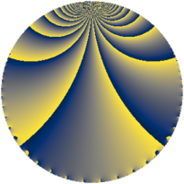# Properties

 Label 7600.2.a.ceLevel $7600$ Weight $2$ Character orbit 7600.a Self dual yes Analytic conductor $60.686$ Analytic rank $0$ Dimension $4$ CM no Inner twists $2$

# Related objects

## Newspace parameters

 Level: $$N$$ $$=$$ $$7600 = 2^{4} \cdot 5^{2} \cdot 19$$ Weight: $$k$$ $$=$$ $$2$$ Character orbit: $$[\chi]$$ $$=$$ 7600.a (trivial)

## Newform invariants

 Self dual: yes Analytic conductor: $$60.6863055362$$ Analytic rank: $$0$$ Dimension: $$4$$ Coefficient field: $$\Q(\sqrt{2}, \sqrt{5})$$ Defining polynomial: $$x^{4} - 6 x^{2} + 4$$ Coefficient ring: $$\Z[a_1, a_2, a_3]$$ Coefficient ring index: $$2^{2}$$ Twist minimal: no (minimal twist has level 380) Fricke sign: $$-1$$ Sato-Tate group: $\mathrm{SU}(2)$

## $q$-expansion

Coefficients of the $$q$$-expansion are expressed in terms of a basis $$1,\beta_1,\beta_2,\beta_3$$ for the coefficient ring described below. We also show the integral $$q$$-expansion of the trace form.

 $$f(q)$$ $$=$$ $$q + \beta_{1} q^{3} + ( -\beta_{1} - \beta_{3} ) q^{7} + \beta_{2} q^{9} +O(q^{10})$$ $$q + \beta_{1} q^{3} + ( -\beta_{1} - \beta_{3} ) q^{7} + \beta_{2} q^{9} + ( 3 + \beta_{2} ) q^{11} + ( 2 \beta_{1} - \beta_{3} ) q^{13} -2 \beta_{3} q^{17} + q^{19} + ( -2 - 2 \beta_{2} ) q^{21} + ( 3 \beta_{1} + \beta_{3} ) q^{23} + ( -\beta_{1} + \beta_{3} ) q^{27} + 2 \beta_{2} q^{29} + 4 q^{31} + ( 5 \beta_{1} + \beta_{3} ) q^{33} + 3 \beta_{1} q^{37} + ( 7 + \beta_{2} ) q^{39} -6 q^{41} + ( -3 \beta_{1} - 3 \beta_{3} ) q^{43} + ( -3 \beta_{1} - 3 \beta_{3} ) q^{47} + q^{49} + ( 2 - 2 \beta_{2} ) q^{51} -3 \beta_{1} q^{53} + \beta_{1} q^{57} + ( 6 + 2 \beta_{2} ) q^{59} + ( -5 + 3 \beta_{2} ) q^{61} + ( -3 \beta_{1} + \beta_{3} ) q^{63} -3 \beta_{3} q^{67} + ( 8 + 4 \beta_{2} ) q^{69} + ( 6 - 2 \beta_{2} ) q^{71} -6 \beta_{1} q^{73} + ( -6 \beta_{1} - 2 \beta_{3} ) q^{77} + ( -2 + 6 \beta_{2} ) q^{79} + ( -4 - 3 \beta_{2} ) q^{81} + ( 3 \beta_{1} - 3 \beta_{3} ) q^{83} + ( 4 \beta_{1} + 2 \beta_{3} ) q^{87} + ( -6 - 4 \beta_{2} ) q^{89} + ( 2 - 6 \beta_{2} ) q^{91} + 4 \beta_{1} q^{93} + ( \beta_{1} + 4 \beta_{3} ) q^{97} + ( 5 + 3 \beta_{2} ) q^{99} +O(q^{100})$$ $$\operatorname{Tr}(f)(q)$$ $$=$$ $$4 q + O(q^{10})$$ $$4 q + 12 q^{11} + 4 q^{19} - 8 q^{21} + 16 q^{31} + 28 q^{39} - 24 q^{41} + 4 q^{49} + 8 q^{51} + 24 q^{59} - 20 q^{61} + 32 q^{69} + 24 q^{71} - 8 q^{79} - 16 q^{81} - 24 q^{89} + 8 q^{91} + 20 q^{99} + O(q^{100})$$

Basis of coefficient ring in terms of a root $$\nu$$ of $$x^{4} - 6 x^{2} + 4$$:

 $$\beta_{0}$$ $$=$$ $$1$$ $$\beta_{1}$$ $$=$$ $$\nu$$ $$\beta_{2}$$ $$=$$ $$\nu^{2} - 3$$ $$\beta_{3}$$ $$=$$ $$\nu^{3} - 5 \nu$$
 $$1$$ $$=$$ $$\beta_0$$ $$\nu$$ $$=$$ $$\beta_{1}$$ $$\nu^{2}$$ $$=$$ $$\beta_{2} + 3$$ $$\nu^{3}$$ $$=$$ $$\beta_{3} + 5 \beta_{1}$$

## Embeddings

For each embedding $$\iota_m$$ of the coefficient field, the values $$\iota_m(a_n)$$ are shown below.

For more information on an embedded modular form you can click on its label.

Label $$\iota_m(\nu)$$ $$a_{2}$$ $$a_{3}$$ $$a_{4}$$ $$a_{5}$$ $$a_{6}$$ $$a_{7}$$ $$a_{8}$$ $$a_{9}$$ $$a_{10}$$
1.1
 −2.28825 −0.874032 0.874032 2.28825
0 −2.28825 0 0 0 2.82843 0 2.23607 0
1.2 0 −0.874032 0 0 0 −2.82843 0 −2.23607 0
1.3 0 0.874032 0 0 0 2.82843 0 −2.23607 0
1.4 0 2.28825 0 0 0 −2.82843 0 2.23607 0
 $$n$$: e.g. 2-40 or 990-1000 Significant digits: Format: Complex embeddings Normalized embeddings Satake parameters Satake angles

## Atkin-Lehner signs

$$p$$ Sign
$$2$$ $$-1$$
$$5$$ $$-1$$
$$19$$ $$-1$$

## Inner twists

Char Parity Ord Mult Type
1.a even 1 1 trivial
5.b even 2 1 inner

## Twists

By twisting character orbit
Char Parity Ord Mult Type Twist Min Dim
1.a even 1 1 trivial 7600.2.a.ce 4
4.b odd 2 1 1900.2.a.j 4
5.b even 2 1 inner 7600.2.a.ce 4
5.c odd 4 2 1520.2.d.f 4
20.d odd 2 1 1900.2.a.j 4
20.e even 4 2 380.2.c.a 4
60.l odd 4 2 3420.2.f.a 4

By twisted newform orbit
Twist Min Dim Char Parity Ord Mult Type
380.2.c.a 4 20.e even 4 2
1520.2.d.f 4 5.c odd 4 2
1900.2.a.j 4 4.b odd 2 1
1900.2.a.j 4 20.d odd 2 1
3420.2.f.a 4 60.l odd 4 2
7600.2.a.ce 4 1.a even 1 1 trivial
7600.2.a.ce 4 5.b even 2 1 inner

## Hecke kernels

This newform subspace can be constructed as the intersection of the kernels of the following linear operators acting on $$S_{2}^{\mathrm{new}}(\Gamma_0(7600))$$:

 $$T_{3}^{4} - 6 T_{3}^{2} + 4$$ $$T_{7}^{2} - 8$$ $$T_{11}^{2} - 6 T_{11} + 4$$ $$T_{13}^{4} - 46 T_{13}^{2} + 484$$

## Hecke characteristic polynomials

$p$ $F_p(T)$
$2$ $$T^{4}$$
$3$ $$4 - 6 T^{2} + T^{4}$$
$5$ $$T^{4}$$
$7$ $$( -8 + T^{2} )^{2}$$
$11$ $$( 4 - 6 T + T^{2} )^{2}$$
$13$ $$484 - 46 T^{2} + T^{4}$$
$17$ $$64 - 56 T^{2} + T^{4}$$
$19$ $$( -1 + T )^{4}$$
$23$ $$64 - 56 T^{2} + T^{4}$$
$29$ $$( -20 + T^{2} )^{2}$$
$31$ $$( -4 + T )^{4}$$
$37$ $$324 - 54 T^{2} + T^{4}$$
$41$ $$( 6 + T )^{4}$$
$43$ $$( -72 + T^{2} )^{2}$$
$47$ $$( -72 + T^{2} )^{2}$$
$53$ $$324 - 54 T^{2} + T^{4}$$
$59$ $$( 16 - 12 T + T^{2} )^{2}$$
$61$ $$( -20 + 10 T + T^{2} )^{2}$$
$67$ $$324 - 126 T^{2} + T^{4}$$
$71$ $$( 16 - 12 T + T^{2} )^{2}$$
$73$ $$5184 - 216 T^{2} + T^{4}$$
$79$ $$( -176 + 4 T + T^{2} )^{2}$$
$83$ $$5184 - 216 T^{2} + T^{4}$$
$89$ $$( -44 + 12 T + T^{2} )^{2}$$
$97$ $$3844 - 214 T^{2} + T^{4}$$